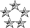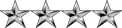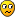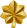## Need help on Set Theory

Mathematics for Computing
NeoPosts: 2642
Joined: Wed Jul 15, 2009 2:07 am
Location: Colombo

### Re: Need help on Set Theory

i expected that nipuna would try to find the answer for the first one.that is why i didnt post a complete answer.
He hasn't done Maths for his A/Ls so he might not be knowing about solving quadratic equations, etc... I think it would be helpful for him to give a detailed description considering that. I know it is not easy to cover all these fundamentals. How do you think?
NeoPosts: 2642
Joined: Wed Jul 15, 2009 2:07 am
Location: Colombo

### Re: Need help on Set Theory

(Q2)

A = {-2, -1, 0, 1, 2, 3 ... N}
B = {-N.....,-2, -1 , 0, 1, 2}

Let's take the first one.
A U B = A

If A U B = A, then B ⊂ A (B must be a subset of A).

To disprove this, we need to find an element x which is in A U B but not in A (A U B ≠ A).

In mathematical language, we write it as below.
A U B ≠ A if ∃x | x ∈A U B AND x ∉ A
This is read as, A U B is not equals to A, if There Exists x, such that x is an element of A U B AND x is not an element of A.

By looking at the elements on these sets, we can easily find -3 which satisfy above condition.
-3 ∈A U B AND -3 ∉ A
∴ A U B ≠ A

With this proof, (a) and (c) are wrong.

Now lets take, A U B = Z
A U B = {-N..., -2, -1, 0, 1, 2, ..... N}
This is the set of all integers denoted as ℤ.
∴ A U B = Z is correct.

Now lets find A∖B (Read as A compliments B). A ∖ B means the set that contains all those elements of A that are not in B.
A∖B = {3, 4, 5, ...., N}
This set can be defined as {n | n ∈ℕ AND n ≥ 3}
∴ A∖B = {n | n ∈ℕ AND n ≥ 3} is correct

With this, only (b) is correct
Nipuna
ModeratorPosts: 2729
Joined: Mon Jan 04, 2010 8:02 pm
Location: Deraniyagala,SRI LANKA

### Re: Need help on Set Theory

Thanks For Helping Me.

Today I've Studied all the Set Algebras which i have in VLE and Memorized them. Now I remember them. (Those 7 Laws with Weird Names)

And I Feel that I have used this one before(in O/L) but I don't remember for whatNow I have an Idea because you Helped me with that.quadeq.PNG (1.7 KiB) Viewed 5020 times
Do you know a Web site with Good Questions like those? Since If there is there would be Answers with them too So I can Easily Study. But BIT Papers Only have Answers not the way of Solving them.

Thanks
NeoPosts: 2642
Joined: Wed Jul 15, 2009 2:07 am
Location: Colombo

### Re: Need help on Set Theory

(Q3)
(i) Wrong. We can't find any element to make the condition (3x > 1 ⋀ 3x < 1) true.
∄x | 3x > 1 ⋀ 3x < 1 (Read as There Doesn't Exist x to fulfil the condition 3x > 1 ⋀ 3x < 1)

(ii) (∃x | 3x > 1) ⋀ (∃x | 3x < 1)

Lets consider ∃x | 3x > 1,

x = 1, 3 > 1
x = 2, 6 > 1
..
..
..
x = N, 3N > 1
We can define this set as {1, 2, 3 ... N}

Now, lets consider ∃x | 3x < 1,

x = 0, 0 < 1
x = -1, -3 < 1
..
..
..
x = -N, -3N < 1
We can define this set as {-N, ....-2, -1, 0}

(∃x | 3x > 1) ⋀ (∃x | 3x < 1) means {1, 2, 3 ... N} ∩ {-N, ....-2, -1, 0} = ∅ (Empty set)

That means we can't find any element to satisfy this condition.

∴ (∃x | 3x > 1) ⋀ (∃x | 3x < 1) is false.

So this is incorrect.

(iii) (∃x | 3x > 1) ⋁ (∃x | 3x < 1)
We already know both sides of these sets.
(∃x | 3x > 1) ⋁ (∃x | 3x < 1) means {1, 2, 3 ... N} ∪ {-N, ....-2, -1, 0} = ℤ (Set of integers)

So this is not an empty set. (∃x | 3x > 1) ⋁ (∃x | 3x < 1) is true.

∴ This is correct.
NeoPosts: 2642
Joined: Wed Jul 15, 2009 2:07 am
Location: Colombo

### Re: Need help on Set Theory

(Q4)
(i) ∀x, x2 + x ≥ 2 ∧ x2 ≥ 1

Our universal set is the set of positive integers. That is {1, 2, 3, 4, 5..... N}
The smallest element is 1.

12 + 1 = 2
22 + 2 = 6 ≥ 2
...
...
This is clearly increasing.
x2 + x ≥ 2 is true for all elements in the universal set.

x2 ≥ 1
12 = 1 ≥ 1
22 = 4 ≥ 1
...
...
This is also clearly increasing.
x2 ≥ 1 is true for all elements in the universal set.

When both sides of AND (∧) is true
∀x, x2 + x ≥ 2 ∧ x2 ≥ 1 is true.

(ii) ∀x, x2 + x ≥ 3 ∨ x2 + x = 2
Let's try to find at least one element which doesn't satisfy this.
Let's check x = 1
12 + 1 = 2
2 ≥ 3 is false. So this part is empty.

Since it is OR (∨), we need to see whether the other part is also false. If other part is True, then false OR True = True (Then that element we have chosen satisfy the condition).

12 + 1 = 2 = 2 This part is correct for x = 1.
∴ When x = 1, x2 + x ≥ 3 ∨ x2 + x = 2 is true.

For rest of the element 2, 3 and so on, We can clearly see x2 + x ≥ 3 is true.

∴ ∀x, x2 + x ≥ 3 ∨ x2 + x = 2 is True.

(iii) (∀x, x2 + x > 2) ∨ (∀x, x2 + x = 2)
Let's check the first part.
(∀x, x2 + x > 2)

Let's take x = 1.

12 + 1 = 2
2 > 2 is false.
Since we have found at least one element which doesn't satisfy the condition, we name it as below.
(∀x, x2 + x > 2) is false (Since it fails for x = 1)

Let's check the other side now. (Since it is OR (∨) We can't end up by proving first part is false)
(∀x, x2 + x = 2)
Let's take x = 2
22 + 2 = 6
6 = 2 is also false

∴ (∀x, x2 + x > 2) ∨ (∀x, x2 + x = 2) is false.
Nipuna
ModeratorPosts: 2729
Joined: Mon Jan 04, 2010 8:02 pm
Location: Deraniyagala,SRI LANKA

### Re: Need help on Set Theory

Thanks For Helps Friend.

I made a Simple way to Remember Those weird Set Algebra NamesI A C D I I C

Idempotent Laws

Associative Laws

Commutative Laws

Distributive Laws

Identity Laws

Involution Laws

Complement laws

And De Morgan's Law
NeoPosts: 2642
Joined: Wed Jul 15, 2009 2:07 am
Location: Colombo

### Re: Need help on Set Theory

(Q5)
p = {(a,b), a ≤ b ∧ a, b ∈ℤ}
σ = {(a,b), a ≥ b ∧ a, b ∈ℤ}
p ∪ σ = {(a,b), a, b ∈ℤ}

(a) is correct

ℤ X ℤ (Called direct product of set ℤ) = {(a,b) | a, b ∈ℤ}
p ∪ σ = {(a,b), a, b ∈ℤ} = ℤ X ℤ

(d) is correct
NeoPosts: 2642
Joined: Wed Jul 15, 2009 2:07 am
Location: Colombo

### Re: Need help on Set Theory

I made a Simple way to Remember Those weird Set Algebra NamesWhere is the last D for De Morgan's Law? Should be I A C D I I C D right?
Herath
MajorPosts: 417
Joined: Thu Aug 05, 2010 7:09 pm

### Re: Need help on Set Theory

Neo wrote:
i expected that nipuna would try to find the answer for the first one.that is why i didnt post a complete answer.
He hasn't done Maths for his A/Ls so he might not be knowing about solving quadratic equations, etc... I think it would be helpful for him to give a detailed description considering that. I know it is not easy to cover all these fundamentals. How do you think?
Sorry If I sounded rude. But I did not expect all answers from him. Just wanted him to post the elements of the set B and the final answer which is A and B. I just wanted to make sure that he understood the given notation for the set and few basic things. He might go through the given answer and think he is ok with it. But when in the exam he might get in trouble because he did not tested him self.By the way neo, how do you type these mathematical symbols directly from keyboard. I thought that it was not possible to type directly. Copying and pasting from the character map is going to be a bit mess.
NeoFor the moment, I copy characters straight from those links